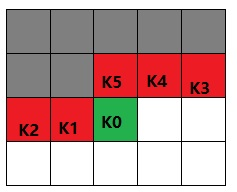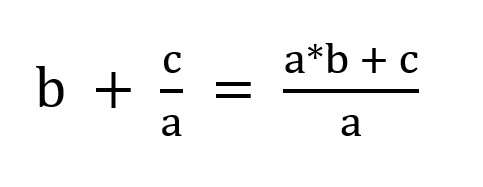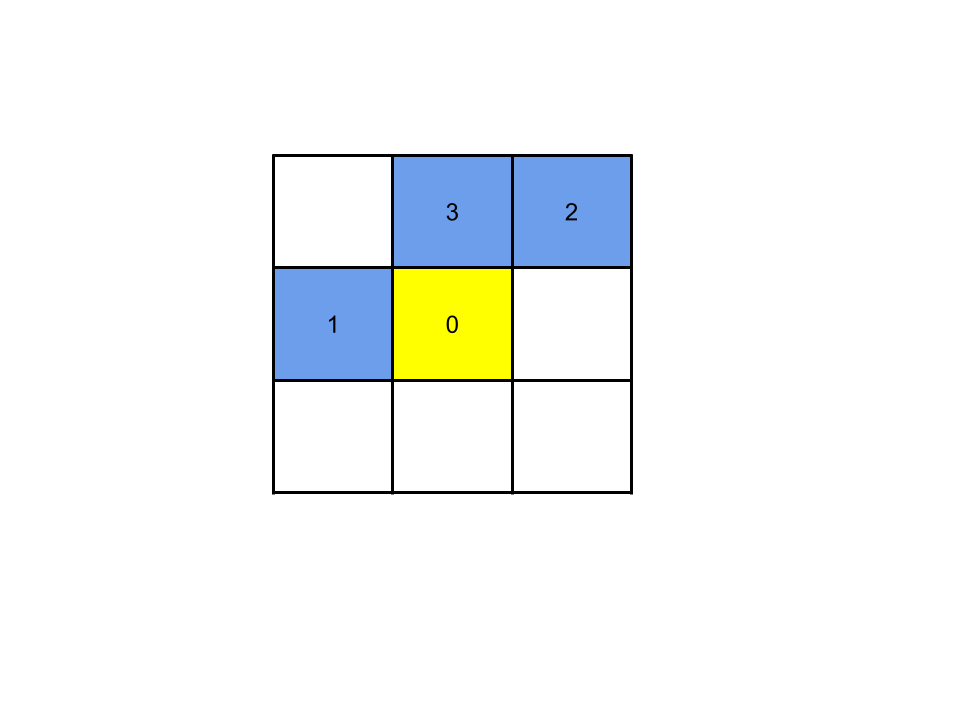🏆2019 力扣杯 - 全国秋季编程大赛5

Problem 1

Problem 2

Problem 3

Problem 4

But... 如果说记录全盘的状态，则状态数会高达 $O(2^{mn})$，在这道题的范围下，状态数堪比棋盘上的麦粒那么多。显然，需要一定技巧来减少需要记录的状态量。P.S.

1. 注意因为动态规划的顺序，横放只可以向左放，竖放只能向上放。

Problem 5

25

### 第一题 主要是拼手速

class Solution {
public:
int game(vector<int>& guess, vector<int>& answer) {
int num = 0;
for(int i=0; i<3; i++)
return num;
}
};


### 第二题 主要是根据公式进行计算，然后化简即可class Solution {
public:
// 传入引用，同步更改传入的实参
void simp(int &a, int &b) {
// __gcd 是 C++ 封装好的求最大公约数的函数，直接调用即可
int g = __gcd(a, b);
a /= g, b /= g;
}
vector<int> fraction(vector<int>& cont) {
int a = cont.back(), b, c = 1;
for(int i=cont.size()-2; i>=0; i--) {
b = cont[i];
if(b == 0) swap(a, c);
else {
int tem = a;
a = a*b + c;
c = tem;
simp(a, c);
}
}
simp(a, c);
return {a, c};
}
};


### 第三题

class Solution {
public:
bool robot(string co, vector<vector<int>>& ob, int x, int y) {
int len = co.size(), a = 0, b = 0, m, n;
// 根据坐标点的 x+y 大小把障碍点排序
sort(ob.begin(), ob.end(), [](vector<int> &a, vector<int> &b) {return a+a < b+b;});
// 通过一个哈希表来记录 记录一轮操作走过的范围
unordered_set<int> hashSet;
hashSet.insert(0);
for(auto &ch:co) {
if(ch == 'U') b++;
else a++;
// 字符串最长不超过 1000
hashSet.insert(a*1000 + b);
}
// 把终点映射到机器人的一轮操作范围内，查看机器人能够经过终点
m = x - (x + y) / len * a;
n = y - (x + y) / len * b;
// 到达不了终点
if(hashSet.find(m*1000 + n) == hashSet.end()) return false;
for(int i=0; i<ob.size(); i++) {
// 终点前面的障碍都碰不到
// 当前障碍的距离大于终点，说明已经到了终点
if(ob[i] + ob[i] > x + y) return true;
m = ob[i] - (ob[i] + ob[i]) / len * a;
n = ob[i] - (ob[i] + ob[i]) / len * b;
// 提前碰到障碍
if(hashSet.find(m*1000 + n) != hashSet.end()) return false;
}
return true;
}
};


### 第四题

class Solution {
public:
bool dfs(int u, vector<bool> &rep, vector<vector<int>> &G, vector<int> &mat) {
for(int i=0; i<G[u].size(); i++) {
int v = G[u][i];
if(!rep[v]) { // 结点如果没有在交替路径中
rep[v] = true;
// 如果当前结点没有匹配,就进行匹配,或者交替路径交换
if(mat[v] == -1 || dfs(mat[v], rep, G, mat)) {
mat[u] = v; // 进行匹配点的记录
mat[v] = u;
return true;
}
}
}
return false;
}
void getRes(int &res, int N, vector<vector<int>> &G) {
// 初始化匹配点都是-1
vector<int> mat(N, -1);
for(int i=0; i<N; i++)
if(mat[i] == -1) {
vector<bool> rep(N, false);
// 每一次增广路径遍历之后,使得匹配点的对数加1
if(dfs(i, rep, G, mat)) res++;
}
}
int domino(int n, int m, vector<vector<int>>& b) {
int N = n*m, u, v, res = 0;
vector<vector<int>> G(N);
vector<vector<bool>> used(n, vector<bool>(m, false));
for(auto &it:b)
used[it][it] = true;

for(int i=0; i<n; i++)
for(int j=0; j<m; j++)
if(!used[i][j]) {
u = i*m+j;
if(j+1 < m && !used[i][j+1]) {
v = i*m + j + 1;
G[u].push_back(v);
G[v].push_back(u);
}
if(i+1 < n && !used[i+1][j]) {
v = i*m + m + j;
G[u].push_back(v);
G[v].push_back(u);
}
}
getRes(res, N, G);
return res;
}
};


### 第五题

class Solution {
public:
const int mod = 1e9 +7;
void dfs(vector<vector<int>>& gr, vector<int>& fa, vector<long long> &res, int x, int y) {
// 增加当前点的每个人以及对应上司的奖金
up(gr, fa, res, x, y);
for(int i=0; i<gr[x].size(); i++)
// 递归遍历每个点
dfs(gr, fa, res, gr[x][i], y);
}
void up(vector<vector<int>>& gr, vector<int>& fa, vector<long long> &res, int cur, int mon) {
// 增加每个人以及对应上司的奖金
while(cur != 0) {
res[cur] = (res[cur] + mon) % mod;
cur = fa[cur];
}
}
vector<int> bonus(int n, vector<vector<int>>& le, vector<vector<int>>& op) {
// 建立基于邻接表的图，因为没有环，所以其实是棵树
vector<vector<int>> graph(n+1);
// 每个节点以及子节点的奖金值
vector<long long> res(n+1, 0);
// 保存每个节点的父节点
vector<int> fa(n+1, 0);
// 建立连接，以及更新父节点
for(auto &it:le) graph[it].push_back(it), fa[it] = it;
vector<int> ret;
for(auto &it:op) {
// 根据命令不同，进行对应操作
if(it == 1) up(graph, fa, res, it, it);
else if(it == 2) dfs(graph, fa, res, it, it);
else ret.push_back(res[it] % mod);
}
return ret;
}
};

17

14

12

10

### 1. 猜数字

class Solution:
def game(self, guess: List[int], answer: List[int]) -> int:
res = 0
for i in range(3):
res += 1
return res


### 2. 分式化简

class Solution:
def fraction(self, cont: List[int]) -> List[int]:
length = len(cont)
res = [cont[length - 1], 1]
for i in range(length - 2, -1, -1):
# 取反
cur = cont[i]
left = cur * res
res = [res + left, res]

return res


### 3. 机器人大冒险

1. 把执行一次命令能走到的点都记录下来，记为 path
2. 把命令中 R 的次数记作 pxU 的次数记作 py

r = min(x // px, y // py)


class Solution:
def robot(self, command: str, obstacles: List[List[int]], x: int, y: int) -> bool:
path = set()

px = 0
py = 0
for c in command:
if c == "R":
px += 1
else:
py += 1

def can_arrive(x, y):
x_round = x // px
y_round = y // py
r = min(x_round, y_round)
lx = x - r * px
ly = y - r * py
if (lx, ly) in path:
return True
else:
return False

if not can_arrive(x, y):
return False

for ob in obstacles:
if ob > x or ob > y:
continue
if can_arrive(ob, ob):
return False

return True


### 4. 覆盖

1. 放骨牌
1. 横着放
2. 竖着放
2. 不放骨牌
1. 不是坏格子，选择不放
2. 是坏格子，不可以放1. 如果格子 0 是一个坏掉的格子：不能放置骨牌
2. 如果格子 0 不是一个坏格子：
1. 如果上侧的格子 3 是一个未被占用的好格子：格子 0 可以竖放骨牌
2. 如果左侧的格子 1 是一个未被占用的好格子：格子 0 可以横放骨牌
3. 如果上侧格子 3 和左侧格子 1 都无法放置骨牌：格子 0 无法放置骨牌

#### 状态表示

• 0 表示格子不放置骨牌
• 1 表示格子放置骨牌

m 个格子就有 $2^m$ 种状态。

#### 状态转移

1. 坏格子：砍掉状态最高位 -> 左移 1 位 -> 最低位置 1
2. 不放骨牌：砍掉状态最高位 -> 左移 1 位
3. 横放骨牌：砍掉状态最高位 -> 左移 1 位 -> 最低两位置 1
4. 竖放骨牌：砍掉状态最高位 -> 左移 1 位 -> 最低位置 1

#### 步骤总结

1. 从左往右、从上往下遍历格子（意图为计算下一个状态 next_dp
2. 在当前格子中遍历所有 $2^m$ 种状态
3. 若状态非法，继续步骤 2
4. 若状态合法，根据状态转移计算出下一个状态 next_state 以及该状态下对应的 dp

#### 实现

class Solution:
def domino(self, n: int, m: int, broken: List[List[int]]) -> int:
# broken 记录
broken_map = dict()
for b in broken:
if b not in broken_map:
broken_map[b] = dict()
broken_map[b][b] = True

"""
是坏格子
"""
def is_broken(a, b):
if a in broken_map and b in broken_map[a]:
return True
else:
return False

"""
获取下一个状态
put: 0-不放，1-横放，2-竖放，3-坏格子
"""
def next_state(state, put):
# 去除高位
state = ~(1 << (m - 1)) & state

if put == 0:
# 不放牌
return state << 1
elif put == 1:
# 横着放
return ((state | 1) << 1) | 1
elif put == 2:
# 竖着放
return (state << 1) | 1
else:
# 坏格子
return (state << 1) | 1

# 创建 dp
dp = [-1] * (1 << m)
dp[(1 << m) - 1] = 0 # 全 1 的置 0

for i in range(n):
for j in range(m):
next_dp = [-1] * (1 << m)
# 遍历所有状态
for state in range(1 << m):
# 非法状态
if dp[state] == -1:
continue

if is_broken(i, j):
# 是坏格子
next_dp[next_state(state, 3)] = max(next_dp[next_state(state, 3)], dp[state]) # 是从 dp 转义还是保持现状
continue

# 不放
next_dp[next_state(state, 0)] = max(next_dp[next_state(state, 0)], dp[state])

# 可以竖放
if i != 0 and (1 << (m - 1)) & state == 0:
next_dp[next_state(state, 2)] = max(next_dp[next_state(state, 2)], dp[state] + 1)

# 可以横放
if j != 0 and 1 & state == 0:
next_dp[next_state(state, 1)] = max(next_dp[next_state(state, 1)], dp[state] + 1)

dp = next_dp

return max(dp)


### 5. 发 LeetCoin

1. 节点的父节点（用于向上更新父节点值）parent
2. 节点和其子节点的 coin 总数 coin
3. 通过操作 2 操作该节点时下发的 coin 数量 children_coin
4. 该节点包含的子节点数 count

#### 操作 1

1. 更新 node 节点的 coin += val
2. 更新其所有父节点的 coin += val

#### 操作 2

1. 更新 node 节点的 coin += all_coin
2. 更新 node 节点的所有父节点 coin += all_coin
3. 更新 node 节点的 children_coin += val

#### 操作 3

node 节点与其子节点的所有 LeetCoin 和为：

node.coin + (node.parent.children_coin * node.count + node.parent.parnet.children_coin * node.count ......)


#### 代码实现

class Node:
def __init__(self):
self.parent = None # 父节点
self.children = [] # 子节点数组
self.coin = 0 # 子树总coin
self.children_coin = 0 # 2 操作分配的 coin（影响到所有子树）
self.count = 1 # 子树节点数

class Solution:
def bonus(self, n: int, leadership: List[List[int]], operations: List[List[int]]) -> List[int]:
# 初始化数据
nodes = dict()
for i in range(1, n + 1):
nodes[i] = Node()

# 记录从属关系
wnode = nodes[worker]
lnode.children.append(wnode)
wnode.parent = lnode

"""
计算 count
"""
def cal_count(root):
for child in root.children:
cal_count(child)
root.count += child.count
return

"""
更新父项
"""
def update_parents(node, coin):
while node:
node.coin += coin
node = node.parent

"""
获取查询结果
"""
def get_res(node):
res = node.coin
node_count = node.count
parent = node.parent
while parent:
res += parent.children_coin * node_count
parent = parent.parent
return res

# 计算 count
cal_count(nodes)

res = []
for op in operations:
worker = op
if op == 1:
# 操作 1：个人发放
nodes[worker].coin += op
update_parents(nodes[worker].parent, op) # 更新它的所有父节点
elif op == 2:
# 操作 2：团队发放
all_coin = nodes[worker].count * op
# 当前节点加上数量
nodes[worker].children_coin += op
nodes[worker].coin += all_coin
# 更新父节点
update_parents(nodes[worker].parent, all_coin)
else:
res.append((get_res(nodes[worker])) % (10**9 + 7))
return res

10

6

10

3